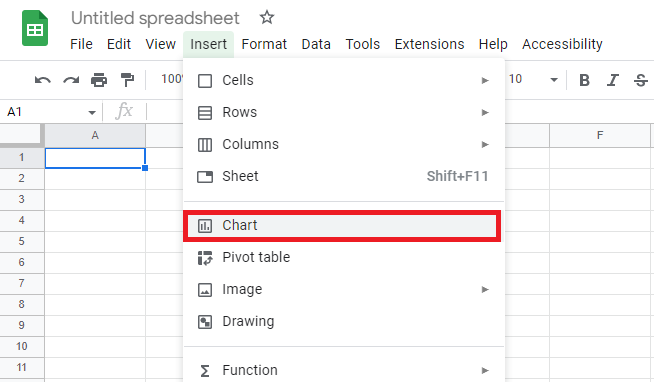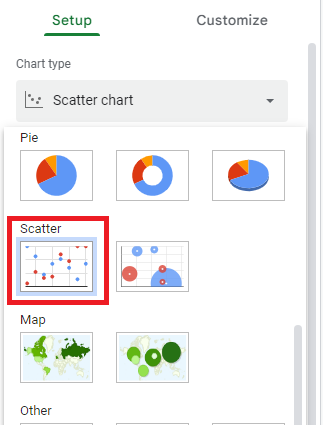However, before we dive into adding equations using the Google Sheets graph maker, let’s define a trendline equation.

## What Is A Trendline Equation?

There are different types of trendlines depending on the chart type and purpose of the trendline. However, this article focuses on the linear trendline. The linear trendline equation is a linear relationship that describes the line that best fits a set of numerical data points. Depending on the nature of a scatter plot, this line should have the shortest distance between each data point and the line itself. The trendline equation is used to define this line. The equation of a linear relationship is y = mx + b. Where x is the independent variable, y is the dependent variable, m is the slope of the line, and b is the y-intercept. Using this equation, you can identify whether or not a trend is present in your data points. Let’s look at how to add this equation to your chart.

To add trendline equations to your chart, you must first have a chart with trendlines. The steps to create one are below:

Firstly, you need to prepare your data. Your number of columns depends on the number of independent variable categories in your data set. You’ll need two columns for a single category— x and y axis.

### Go To Chart

In Google Sheets, you need to select the columns containing your chart, then navigate to Insert > Chart to create your chart.### Data Interpretation

At this point, Google Sheets will create a chart that best suits the data points you’ve provided. In this case, it should automatically create a scatter plot. But if it creates a different chart, simply navigate to Chart Editor > Setup > Scatter plot to create a scatter plot.Now, you should have a raw scatter plot without trendlines. The data points will appear as floaters over spreadsheet cells.

### Chart Editor Customization

Navigate to Chart Editor > Customize > Series, then check the trendline box to add a trendline to your chart.

### Trendline Verification

In the ‘Series’ section under the trendline checkbox, you have options to customize your trendline — line thickness, color, opacity, and more.

### Trendline Visual

Depending on your data points, Google Sheets will display a line (trendline) with the shortest distance between your data points and the line itself.

### Select Use Equation

To add the trendline equation to your scatter plot, navigate to Chart Editor > Customize > Series > Label. In the ‘Label’ dropdown, click on ‘Use Equation’.

### Results

Google Sheets displays the trendline equation at the top of your scatter plot chart. You can add other labels, like chart titles, for better visualization.

## How to Use Excel to Graph an Equation or Function

Excel can be a Google Chart alternative, depending on your project needs. Adding a trend line equation to a scatter plot in Excel is similar to the Google Sheets steps discussed above. However, there are a few differences. But basically, you simply have to create your scatter plot and add the equation. Here’s how you can do so in Excel.

### Creating A Scatterplot

• List your x-axis and y-axis values in different columns. You can do this using the function you want to graph. For example, using the equation, y = 3x + 5 to get the y-axis values.
• Select the columns containing your data.
• Navigate to Insert > Chart.
• Choose the scatter plot from the chart dropdown.

This is where the difference between using Google Sheets and Excel lies.
• Highlight your scatter plot chart.
• Navigate to Chart Design > Add Chart Element.
• Scroll down to ‘Trendline’ and click on it to open the trend line options dropdown.
• Select Linear.
• Click ‘More Trendline Options’ and check the ‘Display Equation On Chart’ checkbox.

### Final Scatterplot With Equation

• Excel will display the equation at the top of your chart. Note that your final scatter plot equation would match the function used for your y-axis values.

## Final Thoughts

The trend line equation enables users to identify trends and outliers. You can go a bit deeper to determine whether or not a trend exists and in what direction.
• If your equation shows a positive slope, i.e., the value of m is positive, there’s a positive relationship between the independent and dependent variables.
• If the value of m is negative, i.e., a negative slope, it represents a negative relationship between variables.
Now that you know how to add equations to graphs in Google Sheets, check out other ways you can improve your chart skills.   Download FusionCharts to try out 100+ charts and 2000+ maps today!

## Take your data visualization to a whole new level

From column to donut and radar to gantt, FusionCharts provides with over 100+ interactive charts & 2,000+ data-driven maps to make your dashboards and reports more insightful

This site uses Akismet to reduce spam. Learn how your comment data is processed.

##### Your next great dashboard starts here

With our interactive and responsive charts, extensive documentation, consistent API, and cross-browser support - delight your customers with kick-ass dashboards